Showing first {{hits.length}} results of {{hits_total}} for {{searchQueryText}}{{hits.length}} results for {{searchQueryText}}

Inga sök resultat

Fractions and binomial coefficients are common mathematical elements with similar characteristics - one number goes on top of another. This article explains how to typeset them in LaTeX. Contents 1 Introduction 2 Displaying fractions 3 Continued fractions 4 Binomial coefficients 5 Reference guide 6 Further reading Introduction Using fractions and binomial coefficients in an expression is straightforward. The binomial coefficient is defined by the next expression: $\binom{n}{k} = \frac{n!}{k!(n-k)!}$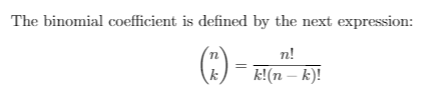For these commands to work you must import the package amsmath by adding the next line to the preamble of your file \usepackage{amsmath}   Open an example in Overleaf Displaying fractions The appearance of the fraction may change depending on the context Fractions can be used alongside the text, for example $$\frac{1}{2}$$, and in a mathematical display style like the one below: $\frac{1}{2}$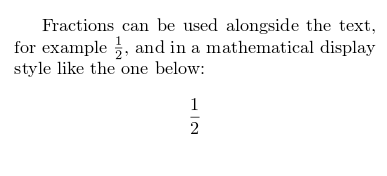As you may have guessed, the command \frac{1}{2} is the one that displays the fraction. The text inside the first pair of braces is the numerator and the text inside the second pair is the denominator. Also, the text size of the fraction changes according to the text around it. You can set this manually if you want. When displaying fractions in-line, for example $$\frac{3x}{2}$$ you can set a different display style: $$\displaystyle \frac{3x}{2}$$. This is also true the other way around $f(x)=\frac{P(x)}{Q(x)} \ \ \textrm{and} \ \ f(x)=\textstyle\frac{P(x)}{Q(x)}$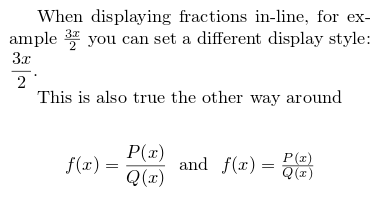The command \displaystyle will format the fraction as if it were in mathematical display mode. On the other side, \textstyle will change the style of the fraction as if it were part of the text.   Open an example in Overleaf Continued fractions The usage of fractions is quite flexible, they can be nested to obtain more complex expressions. The fractions can be nested $\frac{1+\frac{a}{b}}{1+\frac{1}{1+\frac{1}{a}}}$ Now a wild example $a_0+\cfrac{1}{a_1+\cfrac{1}{a_2+\cfrac{1}{a_3+\cdots}}}$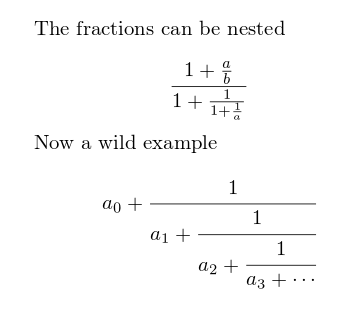The second fraction displayed in the previous example uses the command \cfrac{}{} provided by the package amsmath (see the introduction), this command displays nested fractions without changing the size of the font. Specially useful for continued fractions.   Open an example in Overleaf Binomial coefficients Binomial coefficients are common elements in mathematical expressions, the command to display them in LaTeX is very similar to the one used for fractions. The binomial coefficient is defined by the next expression: $\binom{n}{k} = \frac{n!}{k!(n-k)!}$ And of course this command can be included in the normal text flow $$\binom{n}{k}$$.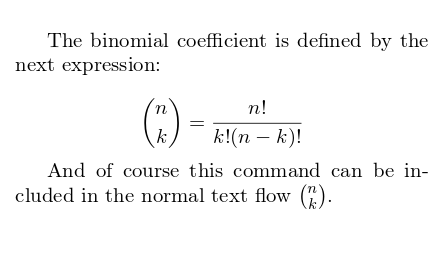As you see, the command \binom{}{} will print the binomial coefficient using the parameters passed inside the braces.   Open an example in Overleaf Reference guide A slightly different and more complex example of continued fractions Final example \newcommand*{\contfrac}{% { \rlap{$\dfrac{1}{\phantom{#1}}$}% \genfrac{}{}{0pt}{0}{}{$1 +$2}% } } $a_0 + \contfrac{a_1}{ \contfrac{a_2}{ \contfrac{a_3}{ \genfrac{}{}{0pt}{0}{}{\ddots} }}}$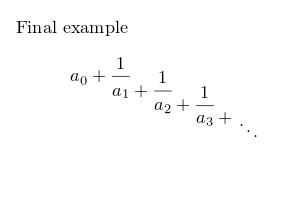Further reading For more information see Mathematical expressions Subscripts and superscripts Brackets and Parentheses Aligning equations with amsmath Operators Mathematical fonts The not so short introduction to LaTeX2ε